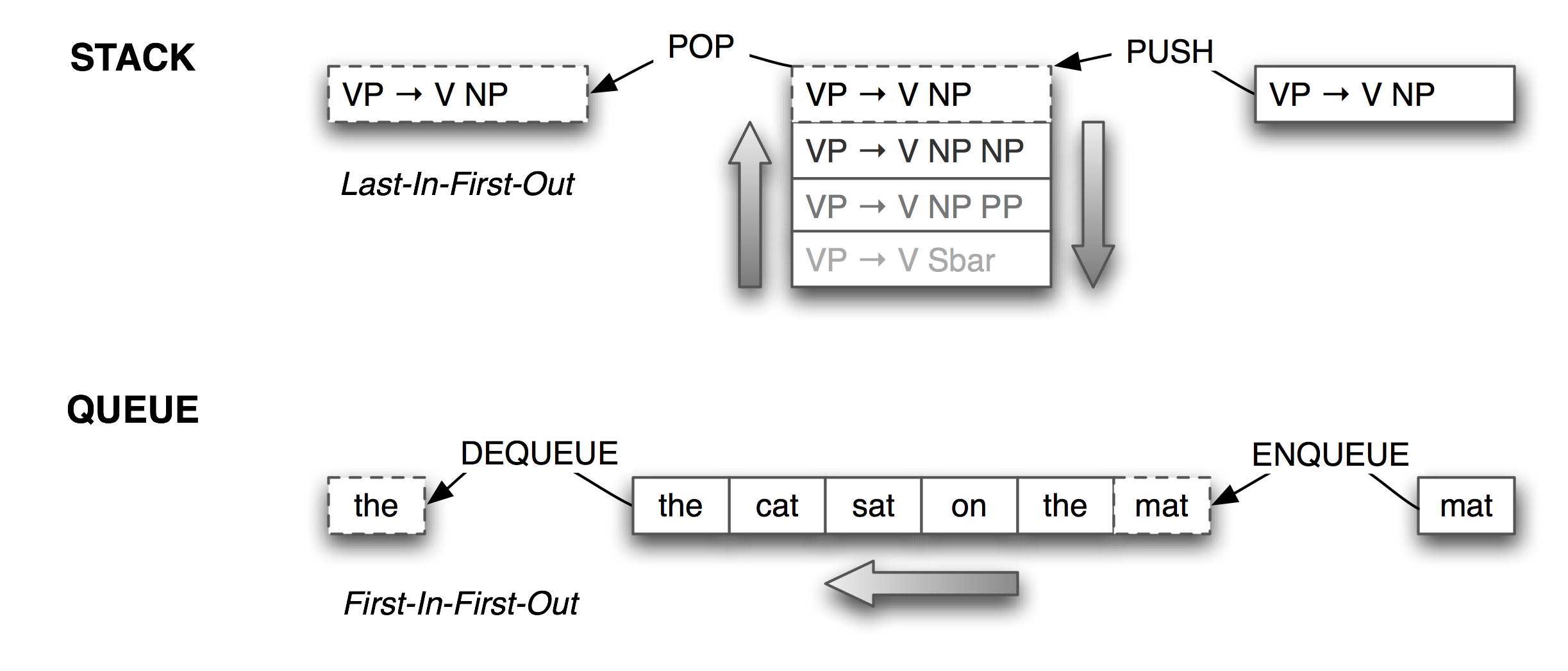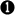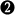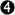# 4   Writing Structured Programs (Extras)

This chapter introduces concepts in algorithms, data structures, program design, and applied Python programming. It also contains review of the basic mathematical notions of set, relation, and function, and illustrates them in terms of Python data structures. It contains many working program fragments that you should try yourself.

## 4.1   The Practice of Software Development

http://www.jwz.org/doc/worse-is-better.html

http://c2.com/cgi/wiki?DontRepeatYourself

import this

• Pages on Python pitfalls.

## 4.2   Abstract Data Types

### Stacks and Queues

Lists are a versatile data type. We can use lists to implement so-called abstract data types such as stacks and queues. A stack is a container that has a last-in-first-out (or LIFO) policy for adding and removing items (see 4.1).Figure 4.1: Stacks and Queues

Stacks are used to keep track of the current context in computer processing of natural languages (and programming languages too). We will seldom have to deal with stacks explicitly, as the implementation of NLTK parsers, treebank corpus readers, (and even Python functions), all use stacks behind the scenes. However, it is important to understand what stacks are and how they work.

 ```def check_parens(tokens): stack = [] for token in tokens: if token == '(': # push stack.append(token) elif token == ')': # pop stack.pop() return stack```
 ```>>> phrase = "( the cat ) ( sat ( on ( the mat )" >>> print check_parens(phrase.split()) ['(', '(']```

Example 4.2 (code_check_parens.py): Figure 4.2: Check whether parentheses are balanced

In Python, we can treat a list as a stack by limiting ourselves to the three operations defined on stacks: append(item) (to push item onto the stack), pop() to pop the item off the top of the stack, and [-1] to access the item on the top of the stack. The program in 4.2 processes a sentence with phrase markers, and checks that the parentheses are balanced. The loop pushes material onto the stack when it gets an open parenthesis, and pops the stack when it gets a close parenthesis. We see that two are left on the stack at the end; i.e. the parentheses are not balanced.

Although the program in 4.2 is a useful illustration of stacks, it is overkill because we could have done a direct count: phrase.count('(') == phrase.count(')'). However, we can use stacks for more sophisticated processing of strings containing nested structure, as shown in 4.3. Here we build a (potentially deeply-nested) list of lists. Whenever a token other than a parenthesis is encountered, we add it to a list at the appropriate level of nesting. The stack keeps track of this level of nesting, exploiting the fact that the item at the top of the stack is actually shared with a more deeply nested item. (Hint: add diagnostic print statements to the function to help you see what it is doing.)

 ```def convert_parens(tokens): stack = [[]] for token in tokens: if token == '(': # push sublist = [] stack[-1].append(sublist) stack.append(sublist) elif token == ')': # pop stack.pop() else: # update top of stack stack[-1].append(token) return stack```
 ```>>> phrase = "( the cat ) ( sat ( on ( the mat ) ) )" >>> print convert_parens(phrase.split()) [['the', 'cat'], ['sat', ['on', ['the', 'branch']]]]```

Example 4.3 (code_convert_parens.py): Figure 4.3: Convert a nested phrase into a nested list using a stack

Lists can be used to represent another important data structure. A queue is a container that has a first-in-first-out (or FIFO) policy for adding and removing items (see 4.1). We could use a queue of length n to create all the n-grams of a text. As with stacks, we will seldom have to deal with queues explicitly, as the implementation of NLTK n-gram taggers (5.5) and chart parsers use queues behind the scenes. Here's how queues can be implemented using lists.

 ```>>> queue = ['the', 'cat', 'sat'] >>> queue.append('on') >>> queue.append('the') >>> queue.append('branch') >>> queue.pop(0) 'the' >>> queue.pop(0) 'cat' >>> queue ['sat', 'on', 'the', 'branch']```

Note

The list-based implementation of queues is inefficient for large queues. In such cases, it is better to use Python's built-in support for "double-ended queues", collections.deque.

## 4.3   Chinese and XML

Codecs for processing Chinese text have been incorporated into Python (since version 2.4).

 ```>>> path = nltk.data.find('samples/sinorama-gb.xml') >>> f = codecs.open(path, encoding='gb2312') >>> lines = f.readlines() >>> for l in lines: ... l = l[:-1] ... utf_enc = l.encode('utf8') ... print repr(utf_enc) '' '' '' '\xe7\x94\x9a\xe8\x87\xb3\xe7\x8c\xab\xe4\xbb\xa5\xe4\xba\xba\xe8\xb4\xb5' '' 'In some cases, cats were valued above humans.' ''```

With appropriate support on your terminal, the escaped text string inside the <SENT> element above will be rendered as the following string of ideographs: 甚至猫以人贵.

We can also read in the contents of an XML file using the etree package (at least, if the file is encoded as UTF-8 — as of writing, there seems to be a problem reading GB2312-encoded files in etree).

 ```>>> path = nltk.data.find('samples/sinorama-utf8.xml') >>> from nltk.etree import ElementTree as ET >>> tree = ET.parse(path) >>> text = tree.findtext('sent') >>> uni_text = text.encode('utf8') >>> print repr(uni_text.splitlines()) '\xe7\x94\x9a\xe8\x87\xb3\xe7\x8c\xab\xe4\xbb\xa5\xe4\xba\xba\xe8\xb4\xb5'```

## 4.4   More on Defensive Programming

### The Return Statement

Another aspect of defensive programming concerns the return statement of a function. In order to be confident that all execution paths through a function lead to a return statement, it is best to have a single return statement at the end of the function definition. This approach has a further benefit: it makes it more likely that the function will only return a single type. Thus, the following version of our tag() function is safer. First we assign a default value, then in certain caseswe replace it with a different value. All paths through the function body end at the single return statement.

 ```>>> def tag(word): ... result = 'noun'... if word in ['a', 'the', 'all']:... result = 'det'... return result```

A return statement can be used to pass multiple values back to the calling program, by packing them into a tuple. Here we define a function that returns a tuple consisting of the average word length of a sentence, and the inventory of letters used in the sentence. It would have been clearer to write two separate functions.

 ```>>> def proc_words(words): ... avg_wordlen = sum(len(word) for word in words)/len(words) ... chars_used = ''.join(sorted(set(''.join(words)))) ... return avg_wordlen, chars_used >>> proc_words(['Not', 'a', 'good', 'way', 'to', 'write', 'functions']) (3, 'Nacdefginorstuwy')```

[write version with two separate functions]

## 4.5   Algorithm Design

An algorithm is a "recipe" for solving a problem. For example, to multiply 16 by 12 we might use any of the following methods:

1. Add 16 to itself 12 times over
2. Perform "long multiplication", starting with the least-significant digits of both numbers
3. Look up a multiplication table
4. Repeatedly halve the first number and double the second, 16*12 = 8*24 = 4*48 = 2*96 = 192
5. Do 10*12 to get 120, then add 6*12
6. Rewrite 16*12 as (x+2)(x-2), remember that 14*14=196, and add (+2)(-2) = -4

Each of these methods is a different algorithm, and requires different amounts of computation time and different amounts of intermediate information to store. A similar situation holds for many other superficially simple tasks, such as sorting a list of words.

### Sorting Algorithms

Now, as we saw above, Python provides a built-in function sort() that performs this task efficiently. However, NLTK also provides several algorithms for sorting lists, to illustrate the variety of possible methods. To illustrate the difference in efficiency, we will create a list of 1000 numbers, randomize the list, then sort it, counting the number of list manipulations required.

 ```>>> from random import shuffle >>> a = range(1000) # [0,1,2,...999] >>> shuffle(a) # randomize```

Now we can try a simple sort method called bubble sort, that scans through the list many times, exchanging adjacent items if they are out of order. It sorts the list a in-place, and returns the number of times it modified the list:

 ```>>> from nltk.misc import sort >>> sort.bubble(a) 250918```

We can try the same task using various sorting algorithms. Evidently merge sort is much better than bubble sort, and quicksort is better still.

 ```>>> shuffle(a); sort.merge(a) 6175 >>> shuffle(a); sort.quick(a) 2378```

Readers are encouraged to look at nltk.misc.sort to see how these different methods work. The collection of NLTK modules exemplify a variety of algorithm design techniques, including brute-force, divide-and-conquer, dynamic programming, and greedy search. Readers who would like a systematic introduction to algorithm design should consult the resources mentioned at the end of this tutorial.

### Decorate-Sort-Undecorate

In 4 we saw how to sort a list of items according to some property of the list.

 ```>>> words = 'I turned off the spectroroute'.split() >>> words.sort(cmp) >>> words ['I', 'off', 'spectroroute', 'the', 'turned'] >>> words.sort(lambda x, y: cmp(len(y), len(x))) >>> words ['spectroroute', 'turned', 'off', 'the', 'I']```

This is inefficient when the list of items gets long, as we compute len() twice for every comparison (about 2nlog(n) times). The following is more efficient:

 ```>>> [pair for pair in sorted((len(w), w) for w in words)[::-1]] ['spectroroute', 'turned', 'the', 'off', 'I']```
 ```>>> from timeit import Timer >>> Timer("sorted(words, lambda x, y: cmp(len(y), len(x)))", ... "words='I turned off the spectroroute'.split()").timeit() 8.3548779487609863 >>> Timer("[pair for pair in sorted((len(w), w) for w in words)]", ... "words='I turned off the spectroroute'.split()").timeit() 9.9698889255523682```

MORE: consider what happens as the lists get longer...

Another example: sorting dates of the form "1 Jan 1970"

 ```>>> month_index = { ... "Jan" : 1, "Feb" : 2, "Mar" : 3, "Apr" : 4, ... "May" : 5, "Jun" : 6, "Jul" : 7, "Aug" : 8, ... "Sep" : 9, "Oct" : 10, "Nov" : 11, "Dec" : 12 ... } >>> def date_cmp(date_string1, date_string2): ... (d1,m1,y1) = date_string1.split() ... (d2,m2,y2) = date_string2.split() ... conv1 = y1, month_index[m1], d1 ... conv2 = y2, month_index[m2], d2 ... return cmp(a2, b2) >>> sort(date_list, date_cmp)```

The comparison function says that we compare two times of the form ('Mar', '2004') by reversing the order of the month and year, and converting the month into a number to get ('2004', '3'), then using Python's built-in cmp function to compare them.

Now do this using decorate-sort-undecorate, for large data size

Time comparison

### Brute Force

Wordfinder Puzzle

Here we will generate a grid of letters, containing words found in the dictionary. First we remove any duplicates and disregard the order in which the lexemes appeared in the dictionary. We do this by converting it to a set, then back to a list. Then we select the first 200 words, and then only keep those words having a reasonable length.

 ```>>> words = list(set(lexemes)) >>> words = words[:200] >>> words = [w for w in words if 3 <= len(w) <= 12]```

Now we generate the wordfinder grid, and print it out.

 ```>>> from nltk.misc.wordfinder import wordfinder >>> grid, used = wordfinder(words) >>> for i in range(len(grid)): ... for j in range(len(grid[i])): ... print grid[i][j], ... print O G H K U U V U V K U O R O V A K U N C K Z O T O I S E K S N A I E R E P A K C I A R A A K I O Y O V R S K A W J K U Y L R N H N K R G V U K G I A U D J K V N I I Y E A U N O K O O U K T R K Z A E L A V U K O X V K E R V T I A A E R K R K A U I U G O K U T X U I K N V V L I E O R R K O K N U A J Z T K A K O O S U T R I A U A U A S P V F O R O O K I C A O U V K R R T U I V A O A U K V V S L P E K A I O A I A K R S V K U S A A I X I K O P S V I K R O E O A R E R S E T R O J X O I I S U A G K R O R E R I T A I Y O A R R R A T O O K O I K I W A K E A A R O O E A K I K V O P I K H V O K K G I K T K K L A K A A R M U G E P A U A V Q A I O O O U K N X O G K G A R E A A P O O R K V V P U J E T Z P K B E I E T K U R A N E O A V A E O R U K B V K S Q A V U E C E K K U K I K I R A E K O J I Q K K K```

Finally we generate the words which need to be found.

 ```>>> for i in range(len(used)): ... print "%-12s" % used[i], ... if float(i+1)%5 == 0: print KOKOROPAVIRA KOROROVIVIRA KAEREASIVIRA KOTOKOTOARA KOPUASIVIRA KATAITOAREI KAITUTUVIRA KERIKERISI KOKARAPATO KOKOVURITO KAUKAUVIRA KOKOPUVIRA KAEKAESOTO KAVOVOVIRA KOVAKOVARA KAAREKOPIE KAEPIEVIRA KAPUUPIEPA KOKORUUTO KIKIRAEKO KATAAVIRA KOVOKOVOA KARIVAITO KARUVIRA KAPOKARI KUROVIRA KITUKITU KAKUPUTE KAEREASI KUKURIKO KUPEROO KAKAPUA KIKISI KAVORA KIKIPI KAPUA KAARE KOETO KATAI KUVA KUSI KOVO KOAI```

### Problem Transformation (aka Transform-and-Conquer)

Find words which, when reversed, make legal words. Extremely wasteful brute force solution:

 ```>>> words = nltk.corpus.words.words('en') >>> for word1 in words: ... for word2 in words: ... if word1 == word2[::-1]: ... print word1```

More efficient:

 ```>>> wordlist = set(words) >>> rev_wordlist = set(word[::-1] for word in words) >>> sorted(wordlist.intersection(rev_wordlist)) ['ah', 'are', 'bag', 'ban', 'bard', 'bat', 'bats', 'bib', 'bob', 'boob', 'brag', 'bud', 'buns', 'bus', 'but', 'civic', 'dad', 'dam', 'decal', 'deed', 'deeps', 'deer', 'deliver', 'denier', 'desserts', 'deus', 'devil', 'dial', 'diaper', 'did', 'dim', 'dog', 'don', 'doom', 'drab', 'draw', 'drawer', 'dub', 'dud', 'edit', 'eel', 'eke', 'em', 'emit', 'era', 'ere', 'evil', 'ewe', 'eye', 'fires', 'flog', 'flow', 'gab', 'gag', 'garb', 'gas', 'gel', 'gig', 'gnat', 'god', 'golf', 'gulp', 'gum', 'gums', 'guns', 'gut', 'ha', 'huh', 'keel', 'keels', 'keep', 'knits', 'laced', 'lager', 'laid', 'lap', 'lee', 'leek', 'leer', 'leg', 'leper', 'level', 'lever', 'liar', 'live', 'lived', 'loop', 'loops', 'loot', 'loots', 'mad', 'madam', 'me', 'meet', 'mets', 'mid', 'mood', 'mug', 'nab', 'nap', 'naps', 'net', 'nip', 'nips', 'no', 'nod', 'non', 'noon', 'not', 'now', 'nun', 'nuts', 'on', 'pal', 'pals', 'pan', 'pans', 'par', 'part', 'parts', 'pat', 'paws', 'peek', 'peels', 'peep', 'pep', 'pets', 'pin', 'pins', 'pip', 'pit', 'plug', 'pool', 'pools', 'pop', 'pot', 'pots', 'pup', 'radar', 'rail', 'rap', 'rat', 'rats', 'raw', 'redder', 'redraw', 'reed', 'reel', 'refer', 'regal', 'reined', 'remit', 'repaid', 'repel', 'revel', 'reviled', 'reviver', 'reward', 'rotator', 'rotor', 'sag', 'saw', 'sees', 'serif', 'sexes', 'slap', 'sleek', 'sleep', 'sloop', 'smug', 'snap', 'snaps', 'snip', 'snoops', 'snub', 'snug', 'solos', 'span', 'spans', 'spat', 'speed', 'spin', 'spit', 'spool', 'spoons', 'spot', 'spots', 'stab', 'star', 'stem', 'step', 'stew', 'stink', 'stool', 'stop', 'stops', 'strap', 'straw', 'stressed', 'stun', 'sub', 'sued', 'swap', 'tab', 'tang', 'tap', 'taps', 'tar', 'teem', 'ten', 'tide', 'time', 'timer', 'tip', 'tips', 'tit', 'ton', 'tool', 'top', 'tops', 'trap', 'tub', 'tug', 'war', 'ward', 'warder', 'warts', 'was', 'wets', 'wolf', 'won']```

Observe that this output contains redundant information; each word and its reverse is included. How could we remove this redundancy?

Presorting, sets:

Find words which have at least (or exactly) one instance of all vowels. Instead of writing extremely complex regular expressions, some simple preprocessing does the trick:

 ```>>> words = ["sequoia", "abacadabra", "yiieeaouuu!"] >>> vowels = "aeiou" >>> [w for w in words if set(w).issuperset(vowels)] ['sequoia', 'yiieeaouuu!'] >>> [w for w in words if sorted(c for c in w if c in vowels) == list(vowels)] ['sequoia']```